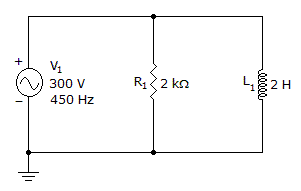# Electronics - Inductors

### Exercise :: Inductors - General Questions

50.

If you wanted to decrease the inductance of a coil, you might increase the:

 A. number of turns B. permeability of the core C. spacing between turns D. area of the coil

Answer: Option C

Explanation:

No answer description available for this question. Let us discuss.

51.

The output phase for a transformer is:

 A. in phase with the input B. out of phase with the input C. dependent on the direction of the primary and secondary windings D. dependent on the frequency of the primary source

Answer: Option C

Explanation:

No answer description available for this question. Let us discuss.

52.

Opposition to current flow without the dissipation of energy is called:

 A. resistance B. inductive reactance C. counter emf D. impedance

Answer: Option B

Explanation:

No answer description available for this question. Let us discuss.

53.

With 100 Vac applied to the primary of a transformer so that it draws 500 mA, what will be the 300 Vac secondary current?

 A. 1500 mA B. 200 mA C. 167 mA D. 150 mA

Answer: Option C

Explanation:

No answer description available for this question. Let us discuss.

54.

Which coil would be used as a step-up transformer primary if coil number 1 has 100 more turns than coil number 2?

 A. The coil windings must be the same. B. coil 1 C. The coil ratio is too small. D. coil 2

Answer: Option D

Explanation:

No answer description available for this question. Let us discuss.

55.

Find IR and IL.A. IR = 50 mA, IL = 109 mA B. IR = 150 mA, IL = 9 mA C. IR = 50 mA, IL = 151 mA D. IR = 150 mA, IL = 53 mA

Answer: Option D

Explanation:

No answer description available for this question. Let us discuss.

56.

When the transformer secondary is not loaded:

 A. secondary voltage increases B. no secondary current flows C. no secondary voltage is present D. secondary current increases

Answer: Option B

Explanation:

No answer description available for this question. Let us discuss.

#### Current Affairs 2021

Interview Questions and Answers